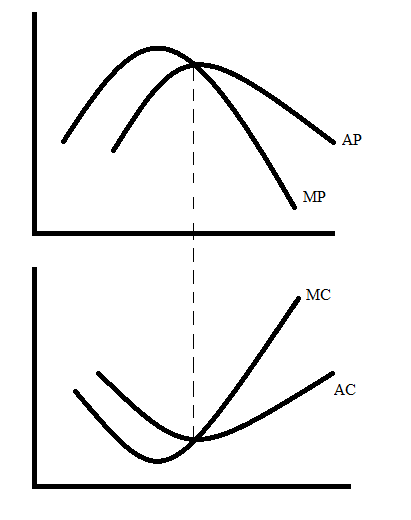# A firm’s marginal product will be at a maximum at which of the following levels of output? Aless than the quantity where average cost is a minimum. Bgreater than the quantity where marginal cost is a minimum. Cgreater than the quantity where average cost is a minimum. Dless than the quantity where marginal cost is a minimum.

Question
10 views
A firm’s marginal product will be at a maximum at which of the following levels of output?

A
less than the quantity where average cost is a minimum.

B
greater than the quantity where marginal cost is a minimum.

C
greater than the quantity where average cost is a minimum.

D
less than the quantity where marginal cost is a minimum.
check_circle

Step 1

To determine the level of output at which a firm's marginal product will be at a maximum.

Step 2

The marginal product is the additional quantity produced when one more worker is hired. Average product is the average number of units produced by the workers. Marginal cost is the additional cost incurred when an additional unit of output is produced. The average cost is the per unit cost of production which can be calculated by dividing the total cost by the output produced.

Step 3

The relationship between the marginal product, average product, marginal cost and the average cost is shown below:The marginal cost curve is just thye mirror image of the marginal product curve. When the average product curve intersects the marginal product curve then the average cost is minimum and the average product curve cuts the marginal product curve when the marginal pr...

### Want to see the full answer?

See Solution

#### Want to see this answer and more?

Solutions are written by subject experts who are available 24/7. Questions are typically answered within 1 hour.*

See Solution
*Response times may vary by subject and question.
Tagged in